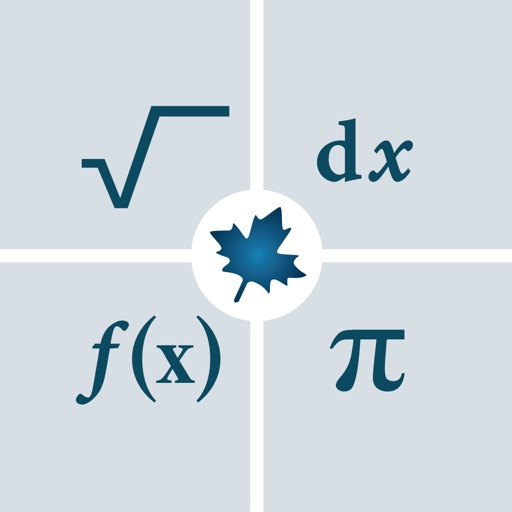## Solve math problems, visualize graphs of 2-D and 3-D expressions and see step-by-step solutions for a wide variety of high-school and university-level questions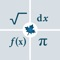# Maple Calculator: Math Helper

by Maplesoft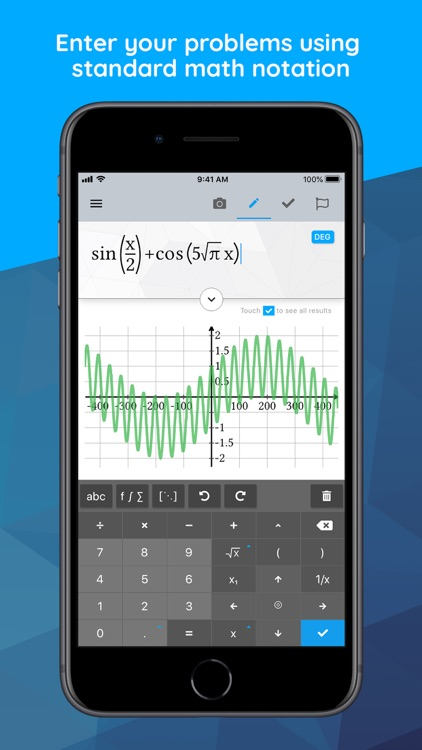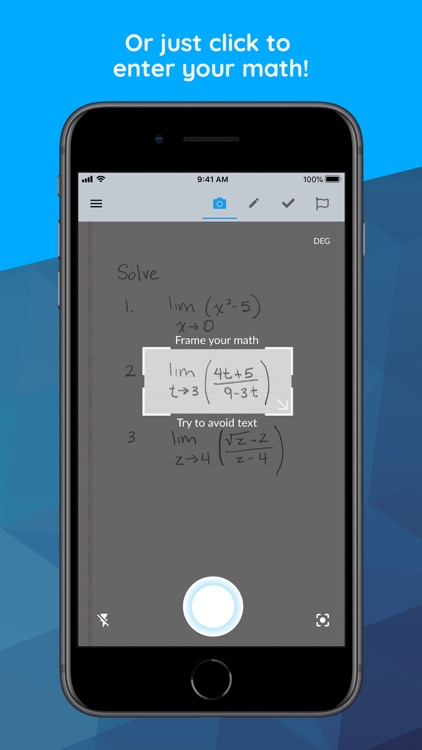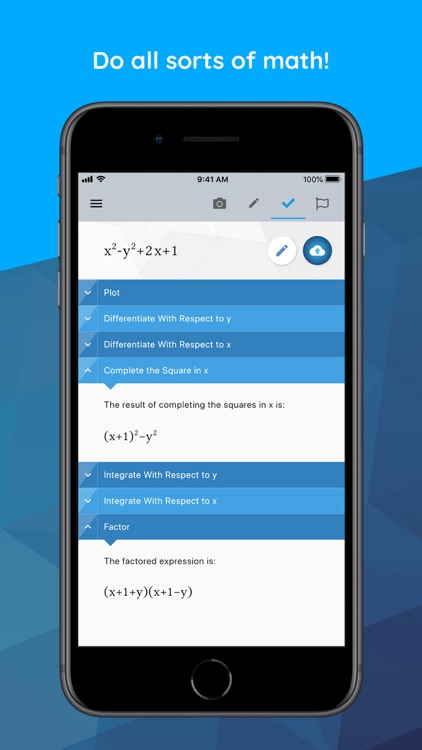Solve math problems, visualize graphs of 2-D and 3-D expressions and see step-by-step solutions for a wide variety of high-school and university-level questions.### App Details

Version
2.6.4
Rating
(363)
Size
68Mb
Genre
Education Utilities
Last updated
June 10, 2021
Release date
September 13, 2019

### App Screenshots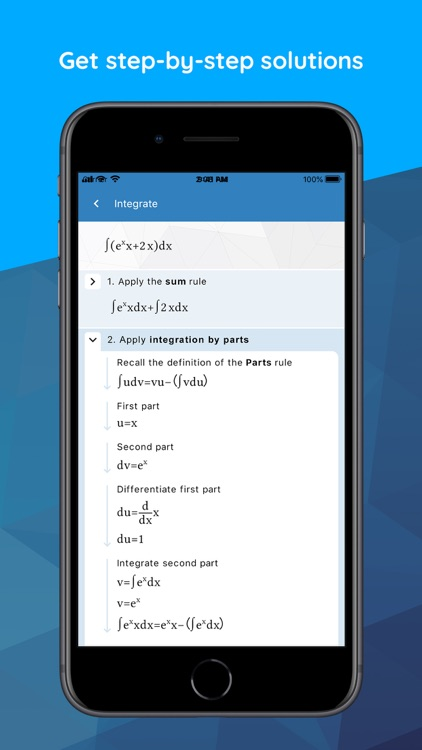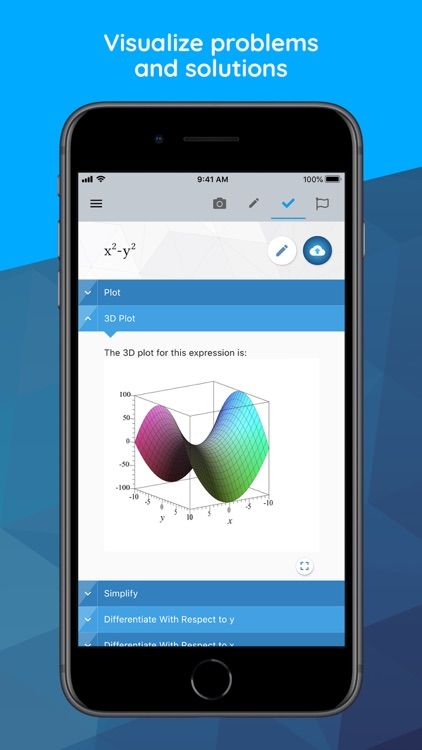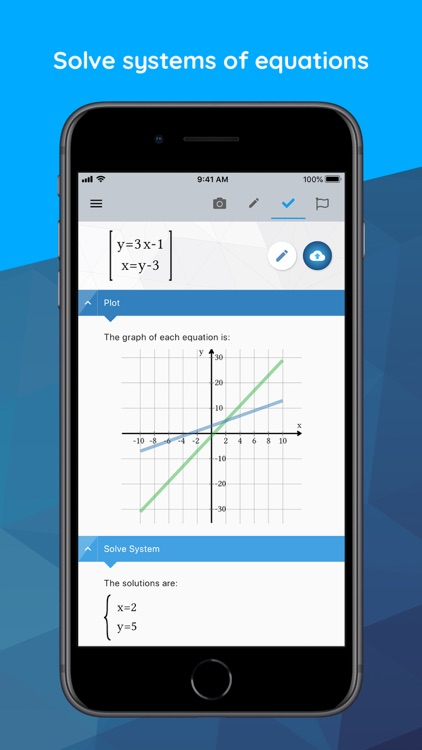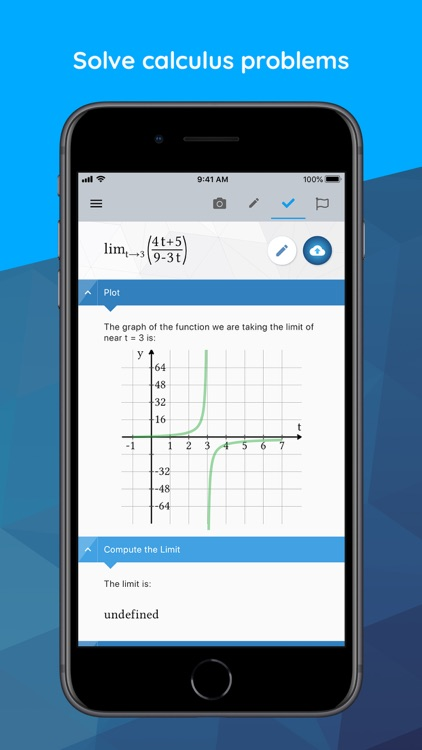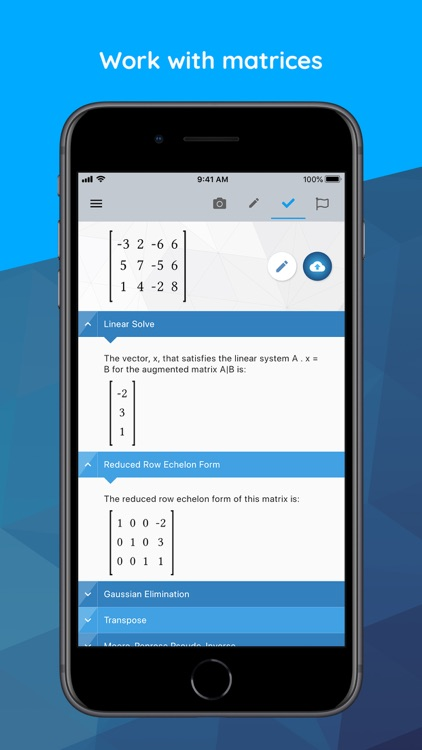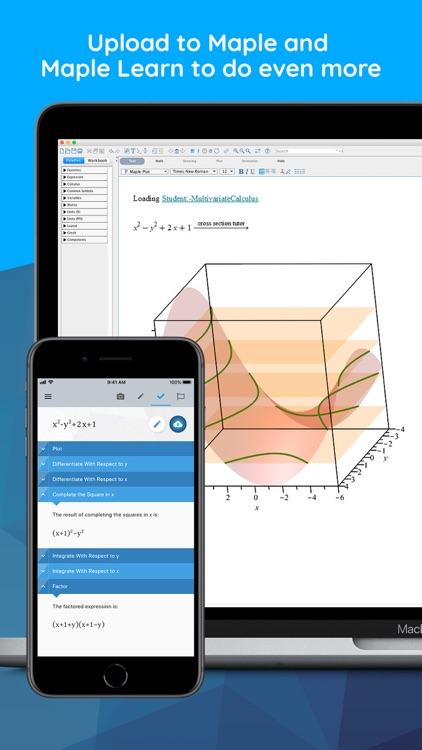### App Store Description

Solve math problems, visualize graphs of 2-D and 3-D expressions and see step-by-step solutions for a wide variety of high-school and university-level questions.

Maple Calculator is a powerful math solver and versatile math learning tool. Whether you are doing simple calculations or working on university-level math problems, Maple Calculator can do it all. Use the calculator to explore 2-D and 3-D graphs, or to see step-by-step solutions to algebra problems, derivative or integrals, matrix manipulations, and so much more!

This versatile app makes it incredibly easy to enter, solve, and visualize mathematical problems from algebra, precalculus, calculus, linear algebra, and differential equations, for free! You can even enter problems using your camera, so you can check your homework with a click of a button. This app is your essential companion to help you learn math, whether you are in a classroom or learning from home.

Key Features

• Just click to enter your math: Enter handwritten and typeset math problems at the click of your camera using powerful AI technology. Or, enter your expression into the calculator using the same mathematical notation your teacher uses – your problem is easy to enter because it “looks right”!
• Do all sorts of math: No matter how you enter your problem, you can find derivatives and integrals, factor polynomials, invert matrices, solve systems of equations, solve ODEs, and much more. The app has the power of the world-leading Maple math engine behind it, so it can do a LOT of math!
• Graph problems and results: See 2-D and 3-D graphs of your expression instantly, and watch how the graph changes as you change the expression. You can zoom in, pan, and even rotate 3-D plots to get a closer look at areas of interest.
• Get step-by-step solutions: In addition to the answer, you get fully worked, step-by-step solutions to a wide variety of math problems, including solving systems of equations; finding limits, derivatives, and, integrals; performing matrix operations, and more!.
• Get answers even when you’re offline: Some aspects of the app require an internet connection, but you can use the powerful graphing calculator anytime!
• Upload calculations directly to Maple Learn: Use the camera to automatically send your handwritten steps to Maple Learn where you can uncover mistakes and share your work with others.
• Avoid transcription errors when using Maple desktop: Avoid the mistakes that can occur when transcribing mathematical expressions into Maple manually, by taking a photo in the app and uploading the results into Maple for further explorations and visualizations
• International language support: Available in English, Spanish, French, German, Russian, Danish, Swedish, Japanese, and Simplified Chinese

Math Capabilities

The Maple Calculator uses the world-renowned Maple mathematics engine, so it can solve many kinds of problems, including:
• Basic Math: Arithmetic, fractions, decimals, integers, factors, square roots, powers
• Algebra: Solving and graphing linear equations, solving and graphing systems of equations, working with polynomials, quadratic equations and functions, logarithmic and exponential functions, trigonometric functions, trigonometric identities
• Precalculus: Graphing, piecewise functions, absolute value, inequalities, implicit functions

Disclaimer:
AppAdvice does not own this application and only provides images and links contained in the iTunes Search API, to help our users find the best apps to download. If you are the developer of this app and would like your information removed, please send a request to [email protected] and your information will be removed.